# GMAT Math : DSQ: Calculating the height of an acute / obtuse triangle

## Example Questions

### Example Question #1 : Triangles

Two sides of a triangle are 6 and 6. What is the height of the triangle?

(1) The third side of the triangle is also 6.

(2) One of the angles of the triangle is.

BOTH statements TOGETHER are sufficient, but NEITHER statement ALONE is sufficient.

Statement (1) and (2) TOGETHER are NOT sufficient to answer the question asked, and additional data are needed.

EACH statement ALONE is sufficient.

Statement (2) ALONE is sufficient, but statement (1) alone is not sufficient.

Statement (1) ALONE is sufficient, but statement (2) alone is not sufficient.

EACH statement ALONE is sufficient.

Explanation:

Statement (1) informs us that the triangle is an equilateral triangle, with all sides equal to 6. Therefore, the height would divide the triangle into two 30-60-90 triangles, which have side lengths in a ratio of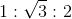. For this triangle the hypotenuse would be 6 and the base would be 3. The height would therefore be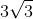. SUFFICIENT

Statement (2) also lets you deduce that the triangle is an equilateral triangle. Since the triangle is either isoscles or equilateral (at least 2 sides are equal), that means that two angles are equal. Therefore, if one angle is, the other two also must be. See above. SUFFICIENT

### Example Question #2 : Triangles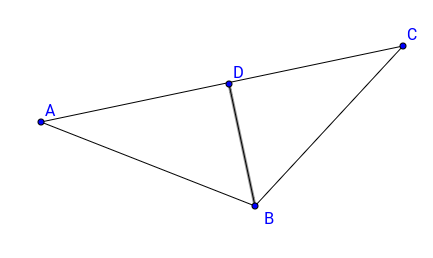Triangle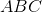has height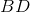. What is the length of?

(1)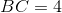.

(2)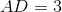.

Statement 2 alone is sufficient.

Each statements alone is sufficient.

Statement 1 alone is sufficient.

Statements 1 and 2 together are not sufficient.

Both statements together are sufficient.

Statements 1 and 2 together are not sufficient.

Explanation:

Since we don't know what type the triangle is, we would not only need information about the lengths of the side but also about the characteristics of the triangle.

Statement 1 gives us the length of a side. However, we can't do anything, since we don't know the length of DC, which would allow us the know BD with the Pythagorean Theorem.

Statement 2 also only gives us information about one side of the triangle. Alone it doesn't allow us to calculate any other length.

Even taken together these statements are insufficient since, we don't know any pair of lengths to use in the Pythagorean Theorem. Even though the triangle looks like a isosceles triangle, it doesn't mean that it is.

### Example Question #3 : Trianglesis a triangle with height. What is the length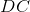?

(1) The triangle has an area ofand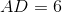.

(2)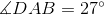and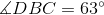.

Statement 2 alone is sufficient.

Statement 1 alone is sufficient.

Both statements together are sufficient.

Each statement alone is sufficient.

Statements 1 and 2 together are insufficient.

Both statements together are sufficient.

Explanation:

To find the length DC, we need to know AD and AC or any of those two provided that ABC is isosceles or equilateral.

Statement 1 tells us the area of the triangle with information about a part of side AC. Since we don't know properties of the triangle, these other lengths can vest many values, just AC can be 12, 24 or 48. Therefore we don't have enough information.

Statement 2 gives us information about angles of the triangle. From what we are told we can see that the triangle is isosceles. Indeed, we know that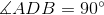since BD is the height. Therefore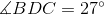. Now, that we know that the triangle is isosceles, we know that AC must be 12, since D is the midpoint of AC. Therefore DC must be 6.

Hence, both statements taken together are sufficient.

Tired of practice problems?

Try live online GMAT prep today.